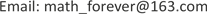1湖南农业大学信息科学技术学院，湖南 长沙

2湖南农业大学农业数学建模与数据处理中心，湖南 长沙1. 引言

2. 数据预处理2.1. 数据来源于模型假设

1) 在脱氧合金化的过程中，不考虑化学反应造成钢水质量的损失。

2) 元素守恒，由于在反应的过程中空气中氧气的影响，质量可以不守恒。

3) 脱氧合金化过程的温度以转炉终点的温度为标准。

2.2. 合金收得率的计算于不同钢种之间差异性检验

Y = C 1 ( W s + ∑ W i ) − C 0 W s ∑ W i δ i

Single factor analysis of varianc
SourceSSDfMSFProb > F
Between groups0.11143440.0278590.920.45
Within groups24.27248040.030189
Total24.38398080.030178

3. 边际影响模型

∂ Y ∂ W s = ( C 1 − C 0 ) ∑ W i δ i

∂ Y ∂ W i = C 1 ∑ W i δ i − ( ( C 1 − C 0 ) W s + C 1 ∑ W i ) δ i ∑ W i δ i ​

Ranking table of marginal impact of factors affecting carbon and manganes

4. 灰色关联度模型

Q o = { Q o ( k ) | k = 1 , 2 , ⋯ , n } = ( Q o ( 1 ) , Q o ( 2 ) , ⋯ , Q o (n))

Q j = { Q j ( k ) | k = 1 , 2 , ⋯ , n } = ( Q j ( 1 ) , Q j ( 2 ) , ⋯ , Q j ( n ) ) , j = 1 , 2 , ⋯ , 23

r i ( k ) = min s min t | Q 0 ( t ) − Q s ( t ) | + ρ max s max t | Q 0 ( t ) − Q s ( t ) | | Q 0 ( t ) − Q i ( t ) | + ρ max s max t | Q 0 ( t ) − Q s ( t ) |

C o r i = 1 n ∑ k = 1 n r i (k)

Ranking of correlation degree of influencing factors of carbon and manganese recover
123456

5. 灰色关联度模型的灵敏度检验

6. 结语